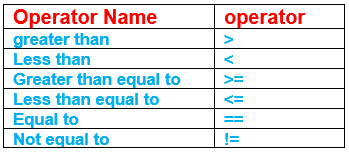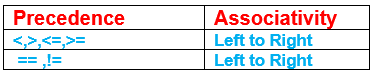# Conditional Statements and Logical Operations in C++

## Conditional Statements and Logical Operations in C++

In this article, I am going to discuss Conditional Statements and Logical Operations in C++ with Examples. As it’s a continuation of the previous discussion of operators. To make our discussion interesting I am going to introduce one of the most important topics in any programming language that is conditional statements along with the logical operators and relational operators. The topics covered in this article are as follows.

1. What are the conditional statements?
2. What are the relational operators?
3. The relation between conditional statements and Relational Operators
4. The relation between conditional statements and Logical Operators
5. If-else condition statement
6. Nested if condition statement
7. short-circuit
8. Switch statement.
9. Assignments
##### Conditional Statements in C++:

The conditional statement comes into the picture when we have choices. To understand better what exactly a conditional statement is let us take an example:

1. Marks: Usually we consider marks above 35 have passed and below 35 has failed. Here we have the choice of pass or fail, which is decided based on marks scored.
2. Offers: If you purchase 4000 and above 10% discount else no discount. Again, here we have the choice of whether to do the shopping for more than 4000 to eligible for a 10% discount.

From the above examples, we can say that usually conditional statements have two states true or false. The true state in programming is 1 and the False state is 0.

##### Example of true state and false state:

If (marks greater than 35)
Pass //This block is an example of a True state
Else
Fail //This block is an example of a False state.

##### Relational Operator in C++

As the name itself suggest Relational operators are used to finding the relation between two operands. Relational Operators in C++ are as follows.Before talking more about Relational operators’ relation with conditional statements, let’s talk about something which we usually discuss in the case of operators.

Yes, you guessed it right…!

Relational operators Precedence and Associativity. If you have skipped the previous tutorial then to know the meaning of operator, precedence, and Associativity please refer to it once.

##### Relational Operator Precedence and Associativity:

Relation operator <, >, <=, >= have same precedence whereas == and != has relatively lower precedence but all relational operators have left to right associativity.Note Arithmetic operators as a higher precedence than Relational operators.

##### The relation between conditional statements and Relational Operators

Usually, conditions are evaluated based on comparison hence both Relational operators and Conditional statements have tight coupling as both go together.

Example: marks>35 here > is a relational operator.

Let us dive into the first conditional statement:

##### Conditional if statement in C++:

In conditional if, the task is performed only if the condition is true. And the false condition is unhandled.

General Syntax:
if(condition){
//logic that needs to implements if true.
}

Example: In the below example print of a happens only when a is 10. Here the other value of a is unhandled. Here == is a relational operator.

```#include <iostream>
using namespace std;
int main ()
{
int a = 10;
if (a == 10)
{
cout << "value of a is 10\n" << endl;
}
return 0;
}
```

Output:##### If-else statement in C++:

As the name suggests here, we handled both the conditions. True and false.

General Syntax:
if(condition)
{
//logic when true;
}
else{
//logic when false;
}

##### Example:
```#include <iostream>
using namespace std;
int main ()
{
int marks;
cin >> marks;
if (marks >= 35)
{
cout << "hey congrats u passed the c++ course" << endl;
}
else
{
cout << "OOPS ..! please retry OOPS once again" << endl;
}
return 0;
}
```

Output:This output shows if the condition fails, else condition gets executed.

When we have multiple conditions to evaluate then we go for Ladder –if statements.

General Syntax:
if(condition){
//implementations
}
else if(condition){
}
else if(condtion){
}
….
…..
else{
//last condtion.
}

##### Example:

To explain these better let’s, take the marks example only.

```#include <iostream>
using namespace std;
int main ()
{
int marks;
cin >> marks;
if (marks >= 35 && marks <= 60)
{
cout << "passed but needs improvement" << endl;
}
else if (marks > 60 && marks <= 75)
{
cout << "good marks try for distinction" << endl;
}
else if (marks > 75 && marks <= 90)
{
cout << "your awesome" << endl;
}
else if (marks > 90 && marks <= 100)
{
cout << "excellent" << endl;
}
else
{
cout << "failed" << endl;
}
return 0;
}
```

Output:Note: don’t really care about the && operators as I am going to explain logical operators soon in this section. There are multiple true conditions in marks so we go for Ladder if.

##### Nested if statement in C++:

Before explaining nested if it is better to explain logical operators. So that you will see how it is used in the program. In the above example in Ladder –if only I have used logical operators.

##### Logical Operators in C++:

Logical operators are used to combining two conditions. In the above ladder if conditions we are used to combining it. Logical Operators are as follows.The truth table for logical operators is explained in my previous article please refer to it.

##### Logical Operator Precedence and Associativity:

Logical-Not (!) has more priority than && and ||##### The relation between conditional statements and Logical Operators

As discussed above logical operators are used when we need to combine two conditions. Hence logical operators have tightly coupled in the case of compound conditional statements.

Example: if(a>10 && b<20) this is the compound conditional statements.

##### Nested if statement:

After a brief introduction of Logical operators, I am re-introducing you to nested if statement. Nested if is nothing but if inside if.

General Syntax:
If(condition)
{
If(condition)
{
//logic
}
}
Else
{
If(condition)
{
//logic;
}
}

##### Example:

To better explain the Nested if let’s modify the same example used in Ladder if to fit to nested if condition.

```#include <iostream>
using namespace std;
int main ()
{
cout << "Please Enter Marks : ";
int marks;
cin >> marks;
if (marks >= 35)
{
cout << "passed step1 cleared" << endl;
if (marks > 60 && marks <= 75)
{
cout << "Good marks try for distinction" << endl;
}

if (marks > 75 && marks <= 90)
{
cout << "Awesome" << endl;
}

if (marks > 90 && marks <= 100)
{
cout << "Excellent" << endl;
}
}
else
{
cout << "Failed" << endl;
}
return 0;
}
```

Output:The reason I modified the ladder if example, 35 and above is considered a pass and distinction, first-class all are part of passing score so I kept these conditions inside if condition. Below 35 is failed so I kept it as it is.

##### Assignments:

Try to solve these questions on your own if you could not able to solve it then look for the assignment solution.

1. program to divide two numbers validates a condition if the denominator is zero.it should pop a message division by zero is impossible.
2. Program to find the maximum of three numbers.
3. Program to Check if the number is odd or even
4. Program to Check if the number is positive or not
5. Program to display the month name. For example, if the user input 11 it should show November.
##### Short Circuit in C++:

It is the optimization technique compiler follows when they are evaluating logical operators. C++ short circuit happens for &&, || operators. In logical And, if the first condition itself is false it won’t check the next condition.

Example:
a=7; b=6; c=8;
if(b>a && ++c>b) here first condition itself is false so it won’t checked ++c>b directly it comes out of the condition. To confirm the same, I have an example program please look into it.

##### Example:
```#include <iostream>
using namespace std;
int main ()
{
int a = 7, b = 6, c = 8;
if (b > a && ++c > b)
{
cout << "wont enter";
}
cout << "value of c expected is 9 as i am incrementing c in if condition " << "but actual value is " << c << endl;
return 0;
}
```

Output:This confirms the second condition is not checked if the checked value would have been 9.

##### The same holds good for Logical OR.

If the first condition itself is true it won’t check the second condition in the case of Logical-OR ||.

Example:

```#include <iostream>
using namespace std;
int main ()
{
int a = 7, b = 6, c = 8;
if (a > b && ++c > b)		//change the logic a>b here a> b so condition is true it wont check second condtion ++c>b
{
cout << "wont enter";
}
cout << "value of c expected is 9 as i am incrementing c in if condition"<< "but actual value is " << c << endl;
return 0;
}
```

Output:##### Switch Statement in C++:

Switch statement you can think like a switchboard where whatever you selected got executed instead of checking all the conditions. It means if you want to turn on the fan you need to switch on the fan switch directly similar way switch works. To achieve the mechanism switch, make use of three keywords.

Switch, case, and break;

###### General Syntax:

Switch(condition/expression)
{
Case 1:
Break;
Case 2:
Break:
Default:
}

Let’s understand how it works. In the above syntax we can see condition/expression if that value is 1 then case 1 gets executed and break encountered so exit the switch condition. If the value is 2 it directed go to case 2 execute it and exit the switch case. If the value is other than 1 and 2 it will execute the default case.

##### Example: simple calculator
```#include <iostream>
using namespace std;

int main ()
{
int a, b, result, choice;
cout << "enter the value of a and b\t" << endl;
cin >> a >> b;
cout <<"enter the choice press 1 for addition 2. for subtraction 3. for multiplication 4. for division" << endl;
cin >> choice;
switch (choice)
{
case 1:
result = a + b;
cout << "sum of two num is\t" << result << endl;
break;
case 2:
result = a - b;
cout << "difference of two num is\t" << result << endl;
break;
case 3:
result = a * b;
cout << "product of two num is\t" << result << endl;
break;
case 4:
result = a / b;
cout << "division of two num is\t" << result << endl;
break;
default:
cout << "you have entered a wrong choice" << endl;
}
}
```

Output:##### Points to Remember:
1. The switch is a branch and control statement
2. Default block is optional
3. If we don’t have a break after each case then all the cases below it get executed.
4. The switch is used for menu-driven programs
5. A switch is more efficient than other conditional statements as it avoids comparison operations.
##### Assignments:

Program to check whether the alphabet is vowel or consonants using switch statements. Try solving assignments on your own if you find any difficulty feel free to reach out to me or you can find the solutions here.

In the next article, I am going to teach you the heart of programming i.e. loops in C++. Here in this article, I try to explain Conditional Statements and Logical Operations in C++ with examples. I hope you enjoy these Conditional Statements and Logical Operations in the C++ article.